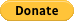# Find Implied Dividend Yield from Option Prices

Dividend estimates are, not only of interest to equity investors who want to earn a steady income, but they also play an important role in derivative pricing for equity forwards, futures, and options including dividend futures and options.

This post shows how to calculate the implied dividend of an option. The implied dividend yield is the yield that makes Put-Call parity true. Option holders typically do not earn dividends, but they closely watch dividend announcements. An European-style option put-call parity yields the following formula to find implied dividend yield from option prices:The formula can be rewritten as:where,

K is the strike price

S is the spot price

T is the time to maturity in years

r is the interest rate to expiry

P(K,T) is the price of a put with strike price K and maturity T

C(K,T) is the price of a call with strike price K and maturity T

q is the implied dividend

If the options are European-style, q can be independent of K.

### Find Implied Dividend Yield from Option Prices

Take the example of YHOO, Yahoo’s option prices for Oct 2014 expiration:

Spot = 40.75

Call = 1.60

Put = 1.46

rate = 0.15%

T = 0.5

Strike = 40.50

Implied Dividend Yield = (-1/0.5) * ln((1.60 – 1.46 + 40.5*e^(-0.015*0.5))/40.75) ~ 2.03%## 2 thoughts on “Find Implied Dividend Yield from Option Prices”

1.Shahram

Thank you for this description.
Is this formula only for European style options?
1.Jish